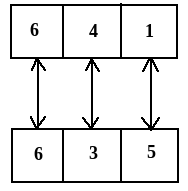# Three positions of a dice are given. Based on them find out which number is found opposite the number 2 in the given cube.Option 1)   6   Option 2)   5   Option 3)   4   Option 4)   1   Option 5)   3

In the above three diagrams,

To find out the opposite faces we will wright all the elements in the clockwise direction starting with the common element in any two figure.

So taking figure (i) & figure (ii) for the above experiment,Here in this diagram we know that 3 is opposite to 4 & 1 is opposite to 5.

Then numbers left = 2 & 6

So we can say that number opposite to 2 = 6

Exams
Articles
Questions